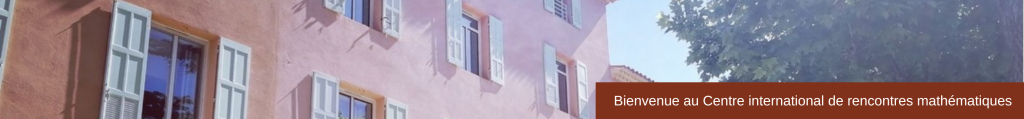CONFERENCE

Prime Numbers and Automatic Sequences : Determinism and Randomness
May 22 – 26, 2017

Scientific & Organizing Committee

Cécile Dartyge (Université de Lorraine)
Michael Drmota (TU Wien)
Bruno Martin (Université du Littoral Côte d’Opale)
Christian Mauduit (Aix-Marseille Université)
Joel Rivat (Aix-Marseille Université)
Thomas Stoll (Université de Lorraine)

The aim of the proposed workshop is to explore further directions for possible collaborative research on the theme of randomness and determinism in the context of analytic number theory (prime numbers, divisors, friable integers, polynomials), theoretical computer science, combinatorics on words and automata theory (automatic sequences, digital functions, complexity measures), and quasi-random sequences.

Through this cross-disciplinary approach, the workshop aims to investigate the interplay between multiplicative properties of integers and deterministic functions such as, for instance, those produced by a dynamical system of zero entropy or those defined by a simple algorithm based on an automaton, and to study correlations, well-distribution measures, normality and orthogonality. A well-known conjecture due to Sarnak says that any bounded sequence of complex numbers produced by a zero topological dynamical system is orthogonal to the Möbius function. Special cases have been treated by Dartyge-Tenenbaum and Mauduit-Rivat (Thue-Morse dynamical system), Green-Tao (translation on a compact nilmanifold) and Bourgain-Sarnak-Ziegler (horocycle flow).

A prominent role in this area of research is played by those functions which rely on the representation of an integer in a number system such as, for instance, the binary expansion of integers. The difficulty of the transition from such a representation of an integer to its multiplicative representation (as a product of prime factors) is at the source of many important open problems in mathematics and computer science.

On the other hand, the study of pseudorandom properties of given deterministic arithmetic sequences, and the conditions under which such deterministic sequences can be regarded as random in certain aspects, is essential for the applications in numerical integration and for the implementation of pseudorandom number generators (pseudorandom measures, discrepancy estimates, correlation measures).

The workshop will bring together researchers of various different areas. The primary goals of the workshop are to promote and encourage cooperation in the areas of:

analytic and probabilistic number theory: prime number theorems in sparse deterministic sequences, prime numbers with digital restrictions, means of related arithmetic functions, Chowla conjecture

theoretical computer science: automatic sequences, Boolean functions, symbolic dynamical systems, computational complexity, Sarnak conjecture

pseudo-random sequences: lacunary sequences, laws of iterated logarithm, low discrepancy point sets

Speakers

Christoph Aistleitner (TU Graz)   Extreme values of the Riemann zeta function via the resonance method
Ramachandran Balasubramanian (IMSc Chennai)   Poisson distribution of a prime counting function corresponding to Elliptic curves
Yann Bugeaud (Université de Strasbourg)   On the representation of real numbers to distinct bases
Lucile Devin (Université Paris-Sud)   Généralisations des biais de Chebyshev
Sary Drappeau (Aix-Marseille Université)    Values of polynomials without large prime factors
Elie Goudout (IMJ – Paris Rive Gauche)    Majoration du nombre d’entiers n  tels que w(n) = k et w(n + 2) = k2
Sigrid Grepstad (University of Linz)   Bounded remainder sets for the discrete and continuous irrational rotation   (pdf)
Gautier Hanna (Aix-Marseille Université)    Blocks of digits of primes
Yining Hu (IMJ – Paris Rive Gauche)    Subword complexity and non-automaticity of certain completely multiplicative functions
Teturo Kamae (Osaka City University)   Selection rules preserving normality
Shanta Laishram (Indian Statistical Institute)   Sums of the digits in bases 2 and 3   (pdf)
Youness Lamzouri (York University)    Large Character Sums   (pdf)
Manfred Madritsch (Université de Lorraine)   The sum-of-digits function of linearly recurrent number systems and almost primes
Alexander Mangerel (University of Toronto)   Some Rigidity Theorems for Multiplicative Functions   (pdf)
James Maynard (Magdalen College, Oxford)    Large gaps between primes in subsets
Clemens Müllner (TU Wien)   Möbius Orthogonality for the Zeckendorf Sum-of-Digits Function   (pdf)
Janos Pintz (Hungarian Academy of Sciences)    The distribution of Zeta-zeros and the remainder term of the prime number theorem   (pdf)
Anne de Roton (Université de Lorraine)    Small sumsets: in continuous versus and discrete setting
Zeev Rudnick (Tel-Aviv University)    Angles of Gaussian primes   (pdf)
Asaki Saito (Future University Hakodate)    Pseudorandom number generator based on the binary expansion of algebraic integers and its p-adic analogue
Adrian Scheerer (TU Graz)   Computable absolutely normal numbers and discrepancies   (pdf)
Cathy Swaenepoel (Aix-Marseille Université)   Digits in finite fields
Jun-Ichi Tamura (Tsuda College)   Convergence theorems of substitutions and Rauzy fractals in the p-adic world
Maciej Ulas (Jagiellonian University)   2-adic valuations of coefficients of certain integer powers of formal power series with {-1; +1} coefficients   (pdf)
Shin-Ichi Yasutomi (Toho University)   Multidimensional p-adic continued fraction algorithms and p-reduced matrices   (pdf)
Zhiwei Wang (Université de Lorraine)   On the largest prime factors of consecutive integers   (pdf)
Máté Wierdl (University of Memphis)   Random differences for arithmetic progressions in the primes   (pdf)